# 味精产品

•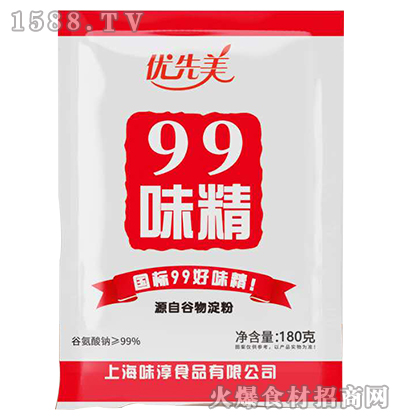我要代理
•我要代理
•我要代理
•我要代理
•我要代理
•我要代理
•我要代理
•我要代理
•我要代理
•我要代理
•我要代理
•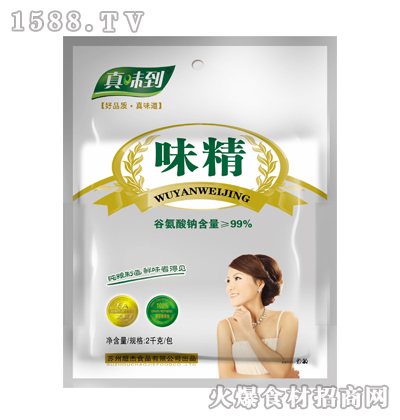我要代理
•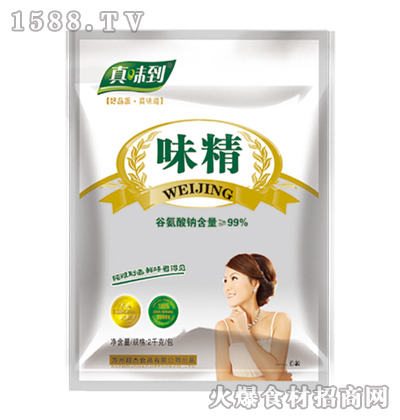我要代理
•我要代理
•我要代理
•我要代理
•我要代理
•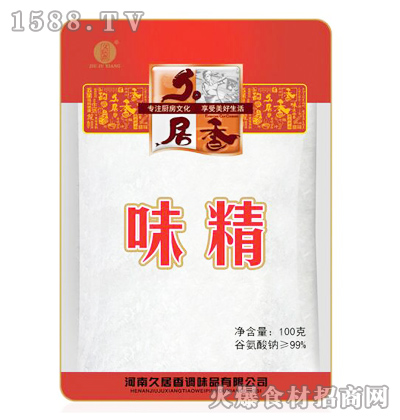我要代理
•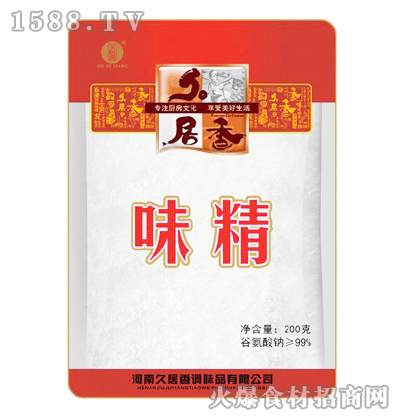我要代理
•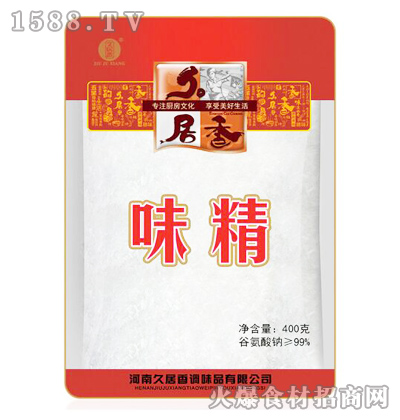我要代理
•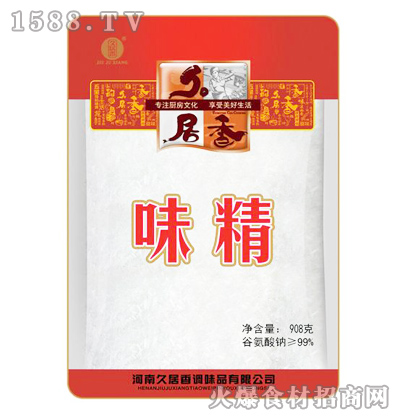我要代理
•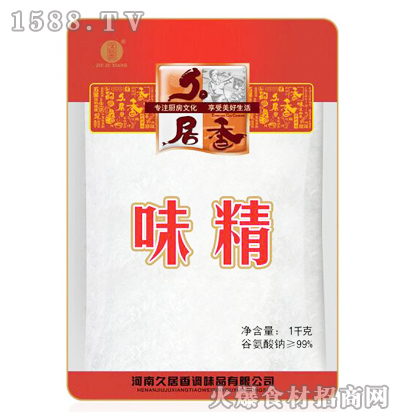我要代理
•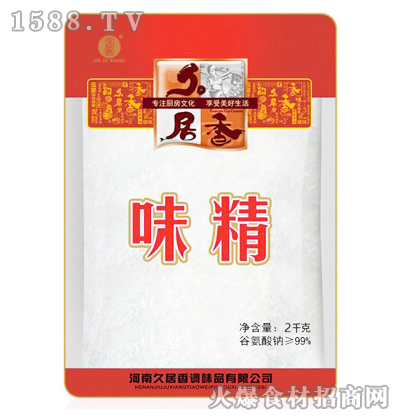我要代理
•我要代理
•我要代理
•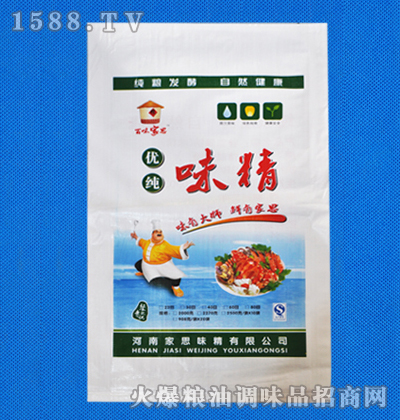我要代理
•我要代理
•我要代理
•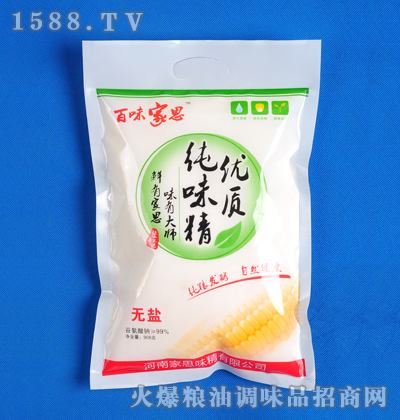我要代理
•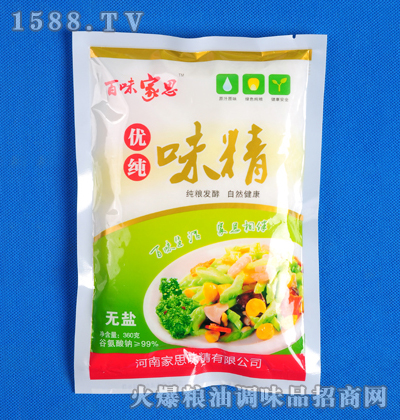我要代理
•我要代理
•我要代理
•我要代理
•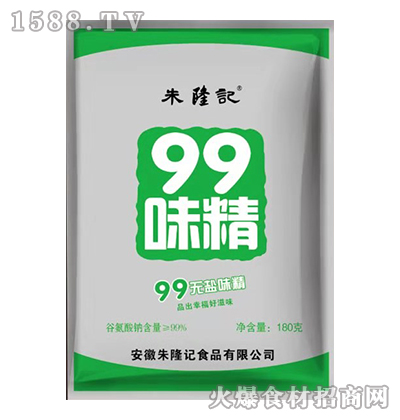我要代理
•我要代理
•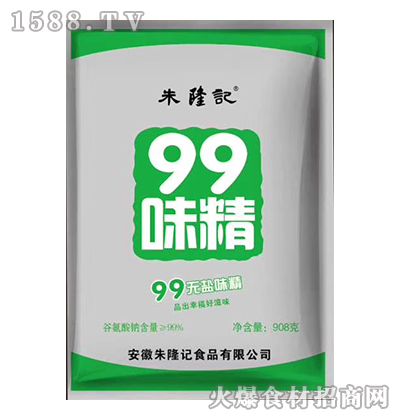我要代理
•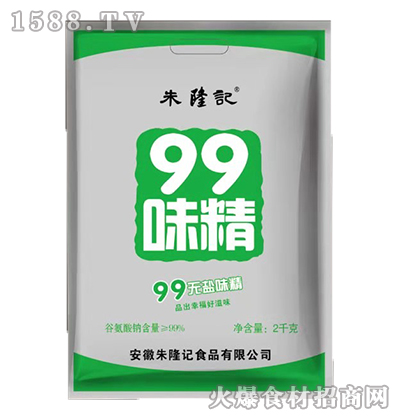我要代理
•我要代理
•我要代理
•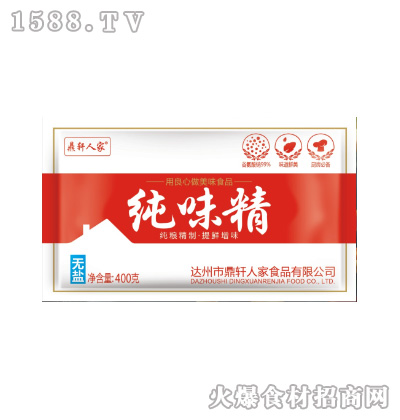我要代理
•我要代理
•我要代理
•我要代理
•我要代理
•我要代理
•我要代理
•我要代理
•我要代理
•我要代理
•我要代理
•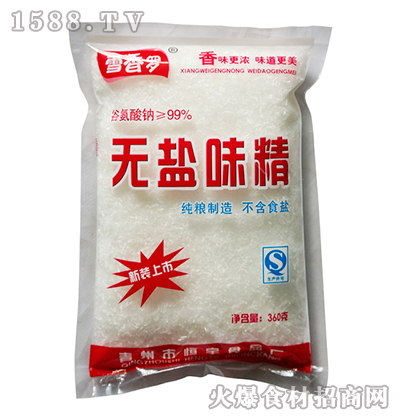我要代理
•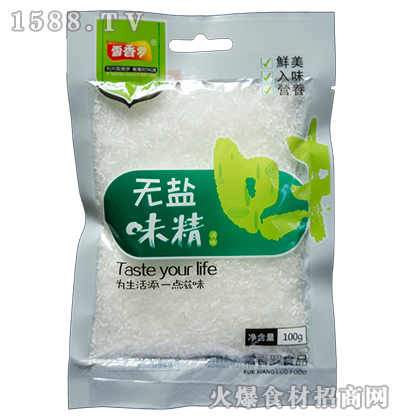我要代理
•我要代理
•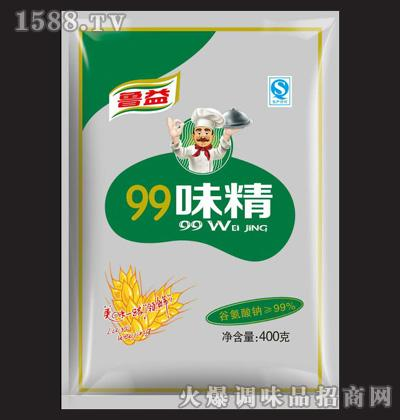我要代理
•我要代理
•我要代理
•我要代理
•我要代理
•我要代理
•我要代理
•我要代理
•我要代理
•我要代理
•我要代理
•我要代理
•我要代理
•我要代理
•我要代理
•我要代理
•我要代理